mscroggs.co.uk
mscroggs.co.uksubscribe

Blog

Archive

Show me a random blog post
▼ show ▼
2017-03-08
This post appeared in issue 05 of Chalkdust. I strongly recommend reading the rest of Chalkdust.
Take a long strip of paper. Fold it in half in the same direction a few times. Unfold it and look at the shape the edge of the paper makes. If you folded the paper $$n$$ times, then the edge will make an order $$n$$ dragon curve, so called because it faintly resembles a dragon. Each of the curves shown on the cover of issue 05 of Chalkdust is an order 10 dragon curve.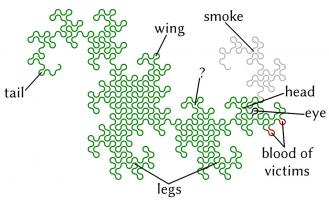Top: Folding a strip of paper in half four times leads to an order four dragon curve (after rounding the corners). Bottom: A level 10 dragon curve resembling a dragon.
The dragon curves on the cover show that it is possible to tile the entire plane with copies of dragon curves of the same order. If any readers are looking for an excellent way to tile a bathroom, I recommend getting some dragon curve-shaped tiles made.
An order $$n$$ dragon curve can be made by joining two order $$n-1$$ dragon curves with a 90° angle between their tails. Therefore, by taking the cover's tiling of the plane with order 10 dragon curves, we may join them into pairs to get a tiling with order 11 dragon curves. We could repeat this to get tilings with order 12, 13, and so on... If we were to repeat this ad infinitum we would arrive at the conclusion that an order $$\infty$$ dragon curve will cover the entire plane without crossing itself. In other words, an order $$\infty$$ dragon curve is a space-filling curve.
Like so many other interesting bits of recreational maths, dragon curves were popularised by Martin Gardner in one of his Mathematical Games columns in Scientific American. In this column, it was noted that the endpoints of dragon curves of different orders (all starting at the same point) lie on a logarithmic spiral. This can be seen in the diagram below.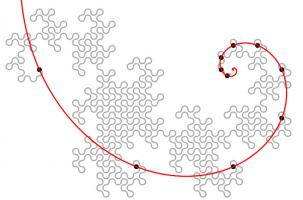The endpoints of dragon curves of order 1 to 10 with a logarithmic spiral passing through them.
Although many of their properties have been known for a long time and are well studied, dragon curves continue to appear in new and interesting places. At last year's Maths Jam conference, Paul Taylor gave a talk about my favourite surprise occurrence of a dragon.
Normally when we write numbers, we write them in base ten, with the digits in the number representing (from right to left) ones, tens, hundreds, thousands, etc. Many readers will be familiar with binary numbers (base two), where the powers of two are used in the place of powers of ten, so the digits represent ones, twos, fours, eights, etc.
In his talk, Paul suggested looking at numbers in base -1+i (where i is the square root of -1; you can find more adventures of i here) using the digits 0 and 1. From right to left, the columns of numbers in this base have values 1, -1+i, -2i, 2+2i, -4, etc. The first 11 numbers in this base are shown below.
 Number in base -1+i Complex number 0 0 1 1 10 -1+i 11 (-1+i)+(1)=i 100 -2i 101 (-2i)+(1)=1-2i 110 (-2i)+(-1+i)=-1-i 111 (-2i)+(-1+i)+(1)=-i 1000 2+2i 1001 (2+2i)+(1)=3+2i 1010 (2+2i)+(-1+i)=1+3i
Complex numbers are often drawn on an Argand diagram: the real part of the number is plotted on the horizontal axis and the imaginary part on the vertical axis. The diagram to the left shows the numbers of ten digits or less in base -1+i on an Argand diagram. The points form an order 10 dragon curve! In fact, plotting numbers of $$n$$ digits or less will draw an order $$n$$ dragon curve.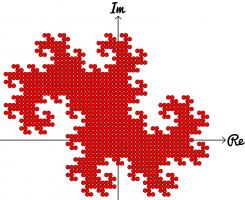Numbers in base -1+i of ten digits or less plotted on an Argand diagram.
Brilliantly, we may now use known properties of dragon curves to discover properties of base -1+i. A level $$\infty$$ dragon curve covers the entire plane without intersecting itself: therefore every Gaussian integer (a number of the form $$a+\text{i} b$$ where $$a$$ and $$b$$ are integers) has a unique representation in base -1+i. The endpoints of dragon curves lie on a logarithmic spiral: therefore numbers of the form $$(-1+\text{i})^n$$, where $$n$$ is an integer, lie on a logarithmic spiral in the complex plane.
If you'd like to play with some dragon curves, you can download the Python code used to make the pictures here.

Similar posts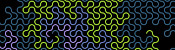Dragon curvesHarriss and other spirals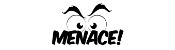MENACE at Manchester Science Festival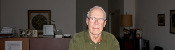The Mathematical Games of Martin Gardner

Comments in green were written by me. Comments in blue were not written by me.

Allowed HTML tags: <br> <a> <small> <b> <i> <s> <sup> <sub> <u> <spoiler> <ul> <ol> <li>
To prove you are not a spam bot, please type "e" then "q" then "u" then "a" then "t" then "i" then "o" then "n" in the box below (case sensitive):
2016-03-30
Take a piece of paper. Fold it in half in the same direction many times. Now unfold it. What pattern will the folds make?
I first found this question in one of Martin Gardner's books. At first, you might that the answer will be simple, but if you look at the shapes made for a few folds, you will see otherwise:
The curves formed are called dragon curves as they allegedly look like dragons with smoke rising from their nostrils. I'm not sure I see the resemblance:
As you increase the order of the curve (the number of times the paper was folded), the dragon curve squiggles across more of the plane, while never crossing itself. In fact, if the process was continued forever, an order infinity dragon curve would cover the whole plane, never crossing itself.
This is not the only way to cover a plane with dragon curves: the curves tessellate.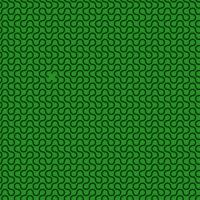When tiled, this picture demonstrates how dragon curves tessellate. For a demonstration, try obtaining infinite lives...
Dragon curves of different orders can also fit together:
To generate digital dragon curves, first notice that an order $$n$$ curve can be made from two order $$n-1$$ curves:
This can easily be seen to be true if you consider folding paper: If you fold a strip of paper in half once, then $$n-1$$ times, each half of the strip will have made an order $$n-1$$ dragon curve. But the whole strip has been folded $$n$$ times, so is an order $$n$$ dragon curve.
Because of this, higher order dragons can be thought of as lots of lower order dragons tiled together. An the infinite dragon curve is actually equivalent to tiling the plane with a infinite number of dragons.
If you would like to create your own dragon curves, you can download the Python code I used to draw them from GitHub. If you are more of a thinker, then you might like to ponder what difference it would make if the folds used to make the dragon were in different directions.

Similar posts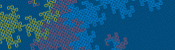Dragon curves IIHarriss and other spirals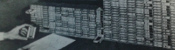MENACE in fiction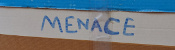Building MENACEs for other games